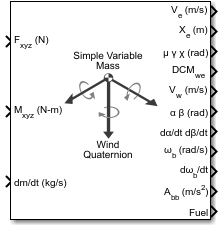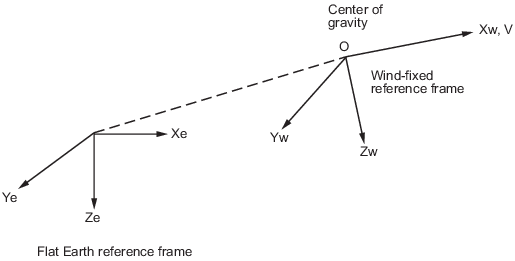# Simple Variable Mass 6DOF Wind (Quaternion)

Implement quaternion representation of six-degrees-of-freedom equations of motion of simple variable mass with respect to wind axes

## Library

Equations of Motion/6DOF

•## Description

The Simple Variable Mass 6DOF Wind (Quaternion) block considers the rotation of a wind-fixed coordinate frame (Xw, Yw, Zw) about an flat Earth reference frame (Xe, Ye, Ze). The origin of the wind-fixed coordinate frame is the center of gravity of the body, and the body is assumed to be rigid, an assumption that eliminates the need to consider the forces acting between individual elements of mass. The flat Earth reference frame is considered inertial, an excellent approximation that allows the forces due to the Earth's motion relative to the “fixed stars” to be neglected.The translational motion of the wind-fixed coordinate frame is given below, where the applied forces [Fx Fy Fz]T are in the wind-fixed frame. Vrew is the relative velocity in the wind axes at which the mass flow ($\stackrel{˙}{m}$) is ejected or added to the body.

`$\begin{array}{l}{\overline{F}}_{w}=\left[\begin{array}{l}{F}_{x}\\ {F}_{y}\\ {F}_{z}\end{array}\right]=m\left({\stackrel{˙}{\overline{V}}}_{w}+{\overline{\omega }}_{w}×{\overline{V}}_{w}\right)+\stackrel{˙}{m}\overline{V}r{e}_{w}\\ {\overline{V}}_{w}=\left[\begin{array}{l}V\\ 0\\ 0\end{array}\right],{\overline{\omega }}_{w}=\left[\begin{array}{l}{p}_{w}\\ {q}_{w}\\ {r}_{w}\end{array}\right]=DM{C}_{wb}\left[\begin{array}{c}{p}_{b}-\stackrel{˙}{\beta }\mathrm{sin}\alpha \\ {q}_{b}-\stackrel{˙}{\alpha }\\ {r}_{b}+\stackrel{˙}{\beta }\mathrm{cos}\alpha \end{array}\right],{\overline{w}}_{b}\left[\begin{array}{l}{p}_{b}\\ {q}_{b}\\ {r}_{b}\end{array}\right]\end{array}$`

The rotational dynamics of the body-fixed frame are given below, where the applied moments are [L M N]T, and the inertia tensor I is with respect to the origin O. Inertia tensor I is much easier to define in body-fixed frame.

`$\begin{array}{l}{\overline{M}}_{b}=\left[\begin{array}{l}L\\ M\\ N\end{array}\right]=I{\stackrel{˙}{\overline{\omega }}}_{b}+{\overline{\omega }}_{b}×\left(I{\overline{\omega }}_{b}\right)+\stackrel{˙}{I}{\overline{\omega }}_{b}\\ I=\left[\begin{array}{lll}{I}_{xx}\hfill & -{I}_{xy}\hfill & -{I}_{xz}\hfill \\ -{I}_{yx}\hfill & {I}_{yy}\hfill & -{I}_{yz}\hfill \\ -{I}_{zx}\hfill & -{I}_{zy}\hfill & {I}_{zz}\hfill \end{array}\right]\end{array}$`

The inertia tensor is determined using a table lookup which linearly interpolates between Ifull and Iempty based on mass (m). While the rate of change of the inertia tensor is estimated by the following equation.

`$\stackrel{˙}{I}=\frac{{I}_{full}-{I}_{empty}}{{m}_{full}-{m}_{empty}}\stackrel{˙}{m}$`

The integration of the rate of change of the quaternion vector is given below.

`$\left[\begin{array}{l}{\stackrel{˙}{q}}_{0}\\ {\stackrel{˙}{q}}_{1}\\ {\stackrel{˙}{q}}_{2}\\ {\stackrel{˙}{q}}_{3}\end{array}\right]=-1/2\left[\begin{array}{llll}0\hfill & p\hfill & q\hfill & r\hfill \\ -p\hfill & 0\hfill & -r\hfill & q\hfill \\ -q\hfill & r\hfill & 0\hfill & -p\hfill \\ -r\hfill & -q\hfill & p\hfill & 0\hfill \end{array}\right]\left[\begin{array}{l}{q}_{0}\\ {q}_{1}\\ {q}_{2}\\ {q}_{3}\end{array}\right]$`

Aerospace Blockset™ uses quaternions that are defined using the scalar-first convention.

## Parameters

### Main

Units

Specifies the input and output units:

UnitsForcesMomentAccelerationVelocityPositionMassInertia
`Metric (MKS)`NewtonNewton meterMeters per second squaredMeters per secondMetersKilogramKilogram meter squared
`English (Velocity in ft/s)`PoundFoot poundFeet per second squaredFeet per secondFeetSlugSlug foot squared
`English (Velocity in kts)`PoundFoot poundFeet per second squaredKnotsFeetSlugSlug foot squared
Mass Type

Select the type of mass to use:

 `Fixed` Mass is constant throughout the simulation. `Simple Variable` Mass and inertia vary linearly as a function of mass rate. `Custom Variable` Mass and inertia variations are customizable.

The `Simple Variable` selection conforms to the previously described equations of motion.

Representation

Select the representation to use:

 `Wind Angles` Use wind angles within equations of motion. `Quaternion` Use quaternions within equations of motion.

The `Quaternion` selection conforms to the previously described equations of motion.

Initial position in inertial axes

The three-element vector for the initial location of the body in the flat Earth reference frame.

Initial airspeed, sideslip angle, and angle of attack

The three-element vector containing the initial airspeed, initial sideslip angle and initial angle of attack.

Initial wind orientation

The three-element vector containing the initial wind angles [bank, flight path, and heading], in radians.

Initial body rotation rates

The three-element vector for the initial body-fixed angular rates, in radians per second.

Initial mass

The initial mass of the rigid body.

Inertia in body axis

A scalar value for the inertia of the body.

Empty mass

A scalar value for the empty mass of the body.

Full mass

A scalar value for the full mass of the body.

Empty inertia matrix

A 3-by-3 inertia tensor matrix for the empty inertia of the body, in body-fixed axes.

Full inertia matrix

A 3-by-3 inertia tensor matrix for the full inertia of the body, in body-fixed axes.

Include mass flow relative velocity

Select this check box to add a mass flow relative velocity port. This is the relative velocity at which the mass is accreted or ablated.

Include inertial acceleration

Select this check box to enable an additional output port for the accelerations in body-fixed axes with respect to the inertial frame. You typically connect this signal to the accelerometer.

### State Attributes

Assign unique name to each state. You can use state names instead of block paths during linearization.

• To assign a name to a single state, enter a unique name between quotes, for example, `'velocity'`.

• To assign names to multiple states, enter a comma-delimited list surrounded by braces, for example, `{'a', 'b', 'c'}`. Each name must be unique.

• If a parameter is empty (`' '`), no name assignment occurs.

• The state names apply only to the selected block with the name parameter.

• The number of states must divide evenly among the number of state names.

• You can specify fewer names than states, but you cannot specify more names than states.

For example, you can specify two names in a system with four states. The first name applies to the first two states and the second name to the last two states.

• To assign state names with a variable in the MATLAB® workspace, enter the variable without quotes. A variable can be a character vector, cell array, or structure.

Position: e.g., {'Xe', 'Ye', 'Ze'}

Specify position state names.

Default value is `''`.

Velocity: e.g., 'V'

Specify velocity state name.

Default value is `''`.

Incidence angle: e.g., 'alpha'

Specify incidence angle state name.

Default value is `''`.

Sideslip angle: e.g., 'beta'

Specify sideslip angle state name.

Default value is `''`.

Wind orientation: e.g., {'mu', 'gamma', 'chi'}

Specify wind orientation state names. This parameter appears if the Representation parameter is set to `Wind Angles`.

Default value is `''`.

Quaternion vector: e.g., {'qr', 'qi', 'qj', 'qk'}

Specify quaternion vector state names. This parameter appears if the Representation parameter is set to `Quaternion`.

Default value is `''`.

Body rotation rates: e.g., {'p', 'q', 'r'}

Specify body rotation rate state names.

Default value is `''`.

Mass: e.g., 'mass'

Specify mass state name.

Default value is `''`.

## Inputs and Outputs

InputDimension TypeDescription

First

VectorContains the three applied forces in wind-fixed axes.

Second

VectorContains the three applied moments in body-fixed axes.

Third

Scalar or vectorContains one or more rates of change of mass.

Fourth (Optional)

VectorContains one or more relative velocities at which the mass is accreted to or ablated from the body in wind axes.
OutputDimension TypeDescription

First

Three-element vector Contains the velocity in the flat Earth reference frame.

Second

Three-element vector Contains the position in the flat Earth reference frame.

Third

Three-element vector Contains the wind rotation angles [bank, flight path, heading], in radians.

Fourth

3-by-3 matrixApplies to the coordinate transformation from flat Earth axes to wind-fixed axes.

Fifth

Three-element vector Contains the velocity in the wind-fixed frame.

Sixth

Two-element vector Contains the angle of attack and sideslip angle, in radians.

Seventh

Two-element vector Contains the rate of change of angle of attack and rate of change of sideslip angle, in radians per second.

Eighth

Three-element vector Contains the angular rates in body-fixed axes, in radians per second.

Ninth

Three-element vector Contains the angular accelerations in body-fixed axes, in radians per second squared.

Tenth

Three-element vector Contains the accelerations in body-fixed axes with respect to body frame.

Eleventh

Scalar elementContains a flag for fuel tank status:
• 1 indicates that the tank is full.

• 0 indicates that the integral is neither full nor empty.

• -1 indicates that the tank is empty.

Twelfth

Three-element vector Contains the accelerations in body-fixed axes with respect to inertial frame (flat Earth). You typically connect this signal to the accelerometer.

## Assumptions and Limitations

The block assumes that the applied forces are acting at the center of gravity of the body.

## References

Stevens, Brian, and Frank Lewis, Aircraft Control and Simulation, Second Edition, John Wiley & Sons, 2003.

Zipfel, Peter H., Modeling and Simulation of Aerospace Vehicle Dynamics. Second Edition, AIAA Education Series, 2007.

## Extended Capabilities

### C/C++ Code GenerationGenerate C and C++ code using Simulink® Coder™.

#### Introduced in R2006a

##### SupportGet trial now